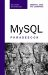Finding the Smallest, Largest, or Average Value in a Column

 # Find the minimum, maximum, and average weights SELECT MIN(wt), AVG(wt), MAX(wt) FROM person;

MySQL provides a variety of functions to perform basic grouping or math on column values. These functions range from grouping functions such as MIN() and MAX(), to simple calculations such as AVG(), all the way to statistical and trigonometric functions.

MIN() and MAX(), respectively, return the minimum and maximum values stored in a given column, while AVG() returns the average of all values in the column as a floating point number.

Interestingly, MIN() and MAX() work with columns that contain text. MIN() returns the value that would come first alphabetically, while MAX() returns the value that would come last alphabetically.

For more information on the various mathematical and grouping functions, see the MySQL online manual, in particular these sections:

• http://dev.mysql.com/doc/refman/5.0/en/group-by-functions-and-modifiers.html

• http://dev.mysql.com/doc/refman/5.0/en/mathematical-functions.htmlMySQL Phrasebook
ISBN: 0672328399
EAN: 2147483647
Year: 2003
Pages: 130

Similar book on Amazon